# ISEE Lower Level Quantitative : Quadrilaterals

## Example Questions

### Example Question #11 : Trapezoids

Angela found the perimeter of the trapezoid below to be 35 cm.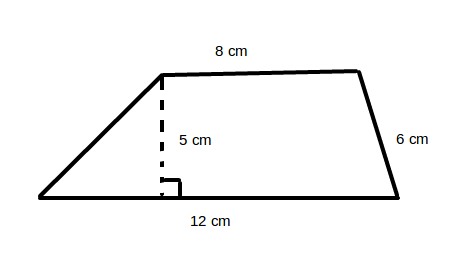What is the length of the missing side?

Possible Answers: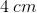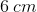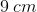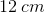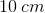Correct answer:Explanation:

To find the perimeter of a trapezoid, add up the lengths of the four sides. In the given trapezoid,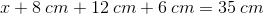To find the missing side, combine like terms and solve for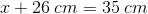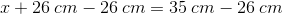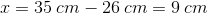The length of the missing side is thus.

### Example Question #12 : Trapezoids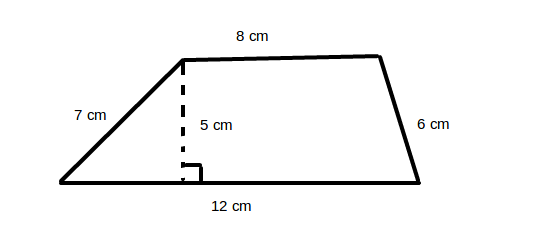What is the perimeter of the above trapezoid?

Possible Answers: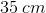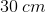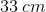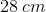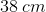Correct answer:Explanation:

To find the perimeter of a trapezoid, add up the lengths of the four sides. In the given trapezoid,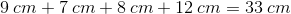### Example Question #1 : Parallelograms

Ted drew the parallelogram shown below.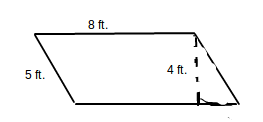What is the perimeter of the parallelogram?

Possible Answers:

12 feet

26 feet

17 feet

40 feet

13 feet

Correct answer:

26 feet

Explanation:

The perimeter of a parallelogram can be found by summing the lengths of each of the sides.  In a parallelogram, opposite sides have the same length.  Therefore, you should use the equation 8 + 8 + 5 + 5 = P.  The perimeter of the parallelogram is 26 feet.

### Example Question #2 : Parallelograms

Guillermo drew the parallelogram below.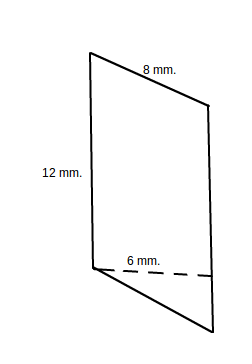What is the perimeter of the parallelogram?

Possible Answers:

26 millimeters

72 millimeters

42 millimeters

40 millimeters

20 millimeters

Correct answer:

40 millimeters

Explanation:

The perimeter of a parallelogram can be found by summing the lengths of each of the sides.  In a parallelogram, opposite sides have the same length.  Therefore, you should use the equation 8 + 8 + 12 + 12 = P.  The perimeter of the parallelogram is 40 feet.

### Example Question #3 : Parallelograms

Find the perimeter of the following parallelogram.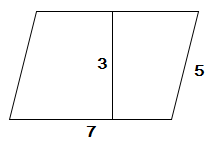Possible Answers:Correct answer:Explanation:

We plug in our values into the equation for the perimeter: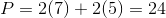### Example Question #4 : Parallelograms

Find the perimeter of the following parallelogram.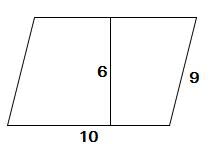Possible Answers: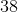Correct answer:Explanation:

We plug in our values into the equation for the perimeter: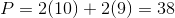### Example Question #5 : Parallelograms

Find the perimeter of the following parallelogram.Possible Answers:Correct answer:Explanation:

We plug in our values into the equation for the perimeter:### Example Question #505 : Measurement & Data

What is the perimeter of the polygon below?Possible Answers: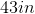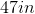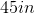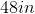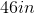Correct answer:Explanation:

To find the area of a perimeter, we add all of the side lengths together.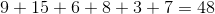### Example Question #506 : Measurement & Data

What is the perimeter of the polygon below?Possible Answers: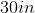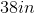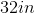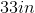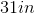Correct answer:Explanation:

To find the area of a perimeter, we add all of the side lengths together.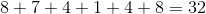### Example Question #507 : Measurement & Data

What is the perimeter of the polygon below?Possible Answers: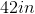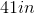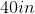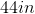Correct answer:Explanation:

To find the area of a perimeter, we add all of the side lengths together.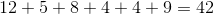### All ISEE Lower Level Quantitative Resources